Chapter 6. Electronic Structure and Periodic Properties of Elements

# 6.1 Electromagnetic Energy

### Learning Objectives

By the end of this section, you will be able to:

• Explain the basic behavior of waves, including travelling waves and standing waves
• Describe the wave nature of light
• Use appropriate equations to calculate related light-wave properties such as period, frequency, wavelength, and energy
• Distinguish between line and continuous emission spectra
• Describe the particle nature of light

The nature of light has been a subject of inquiry since antiquity. In the seventeenth century, Isaac Newton performed experiments with lenses and prisms and was able to demonstrate that white light consists of the individual colors of the rainbow combined together. Newton explained his optics findings in terms of a “corpuscular” view of light, in which light was composed of streams of extremely tiny particles travelling at high speeds according to Newton’s laws of motion. Others in the seventeenth century, such as Christiaan Huygens, had shown that optical phenomena such as reflection and refraction could be equally well explained in terms of light as waves travelling at high speed through a medium called “luminiferous aether” that was thought to permeate all space. Early in the nineteenth century, Thomas Young demonstrated that light passing through narrow, closely spaced slits produced interference patterns that could not be explained in terms of Newtonian particles but could be easily explained in terms of waves. Later in the nineteenth century, after James Clerk Maxwell developed his theory of electromagnetic radiation and showed that light was the visible part of a vast spectrum of electromagnetic waves, the particle view of light became thoroughly discredited. By the end of the nineteenth century, scientists viewed the physical universe as roughly comprising two separate domains: matter composed of particles moving according to Newton’s laws of motion, and electromagnetic radiation consisting of waves governed by Maxwell’s equations. Today, these domains are referred to as classical mechanics and classical electrodynamics (or classical electromagnetism). Although there were a few physical phenomena that could not be explained within this framework, scientists at that time were so confident of the overall soundness of this framework that they viewed these aberrations as puzzling paradoxes that would ultimately be resolved somehow within this framework. As we shall see, these paradoxes led to a contemporary framework that intimately connects particles and waves at a fundamental level called wave-particle duality, which has superseded the classical view.

Visible light and other forms of electromagnetic radiation play important roles in chemistry, since they can be used to infer the energies of electrons within atoms and molecules. Much of modern technology is based on electromagnetic radiation. For example, radio waves from a mobile phone, X-rays used by dentists, the energy used to cook food in your microwave, the radiant heat from red-hot objects, and the light from your television screen are forms of electromagnetic radiation that all exhibit wavelike behavior.

# Waves

A wave is an oscillation or periodic movement that can transport energy from one point in space to another. Common examples of waves are all around us. Shaking the end of a rope transfers energy from your hand to the other end of the rope, dropping a pebble into a pond causes waves to ripple outward along the water’s surface, and the expansion of air that accompanies a lightning strike generates sound waves (thunder) that can travel outward for several miles. In each of these cases, kinetic energy is transferred through matter (the rope, water, or air) while the matter remains essentially in place. An insightful example of a wave occurs in sports stadiums when fans in a narrow region of seats rise simultaneously and stand with their arms raised up for a few seconds before sitting down again while the fans in neighboring sections likewise stand up and sit down in sequence. While this wave can quickly encircle a large stadium in a few seconds, none of the fans actually travel with the wave-they all stay in or above their seats.

Waves need not be restricted to travel through matter. As Maxwell showed, electromagnetic waves consist of an electric field oscillating in step with a perpendicular magnetic field, both of which are perpendicular to the direction of travel. These waves can travel through a vacuum at a constant speed of 2.998 × 108 m/s, the speed of light (denoted by c).

All waves, including forms of electromagnetic radiation, are characterized by, a wavelength (denoted by λ, the lowercase Greek letter lambda), a frequency (denoted by ν, the lowercase Greek letter nu), and an amplitude. As can be seen in Figure 1, the wavelength is the distance between two consecutive peaks or troughs in a wave (measured in meters in the SI system). Electromagnetic waves have wavelengths that fall within an enormous range-wavelengths of kilometers (103 m) to picometers (10−12 m) have been observed. The frequency is the number of wave cycles that pass a specified point in space in a specified amount of time (in the SI system, this is measured in seconds). A cycle corresponds to one complete wavelength. The unit for frequency, expressed as cycles per second [s−1], is the hertz (Hz). Common multiples of this unit are megahertz, (1 MHz = 1 × 106 Hz) and gigahertz (1 GHz = 1 × 109 Hz). The amplitude corresponds to the magnitude of the wave’s displacement and so, in Figure 1, this corresponds to one-half the height between the peaks and troughs. The amplitude is related to the intensity of the wave, which for light is the brightness, and for sound is the loudness.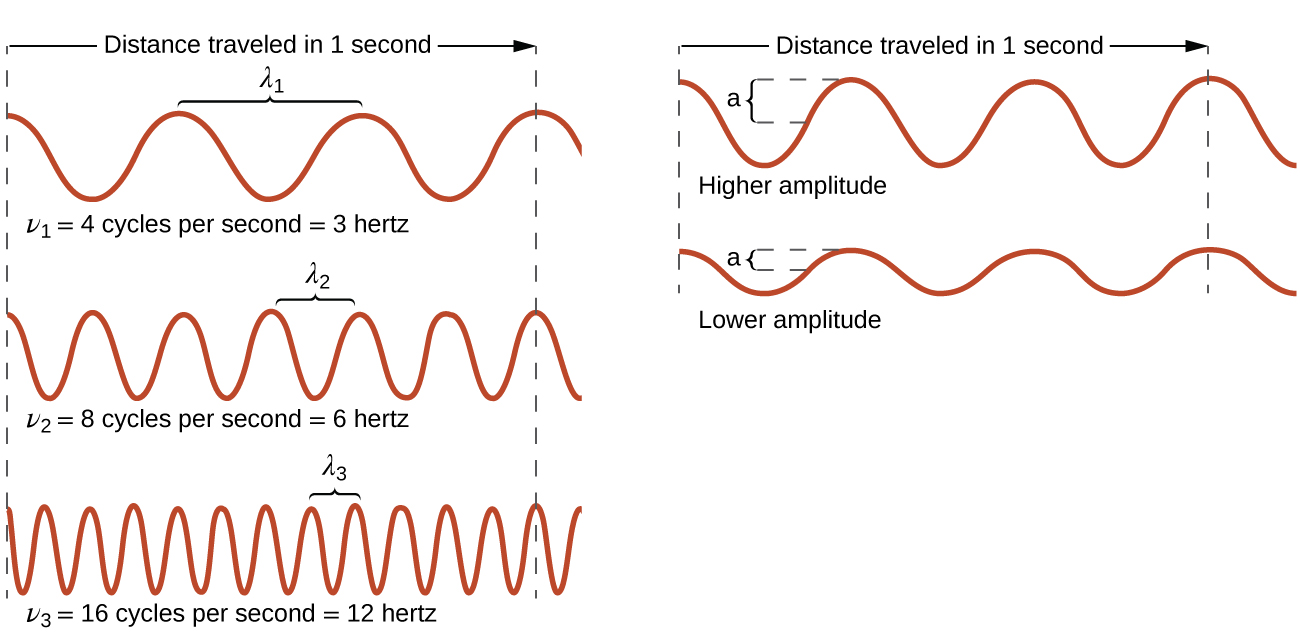Figure 1. One-dimensional sinusoidal waves show the relationship among wavelength, frequency, and speed. The wave with the shortest wavelength has the highest frequency. Amplitude is one-half the height of the wave from peak to trough.

The product of a wave’s wavelength (λ) and its frequency (ν), λν, is the speed of the wave. Thus, for electromagnetic radiation in a vacuum:

$c = 2.998 \times 10^8\text{ms}^{-1} = \lambda\nu$

Wavelength and frequency are inversely proportional: As the wavelength increases, the frequency decreases. The inverse proportionality is illustrated in Figure 2. This figure also shows the electromagnetic spectrum, the range of all types of electromagnetic radiation. Each of the various colors of visible light has specific frequencies and wavelengths associated with them, and you can see that visible light makes up only a small portion of the electromagnetic spectrum. Because the technologies developed to work in various parts of the electromagnetic spectrum are different, for reasons of convenience and historical legacies, different units are typically used for different parts of the spectrum. For example, radio waves are usually specified as frequencies (typically in units of MHz), while the visible region is usually specified in wavelengths (typically in units of nm or angstroms).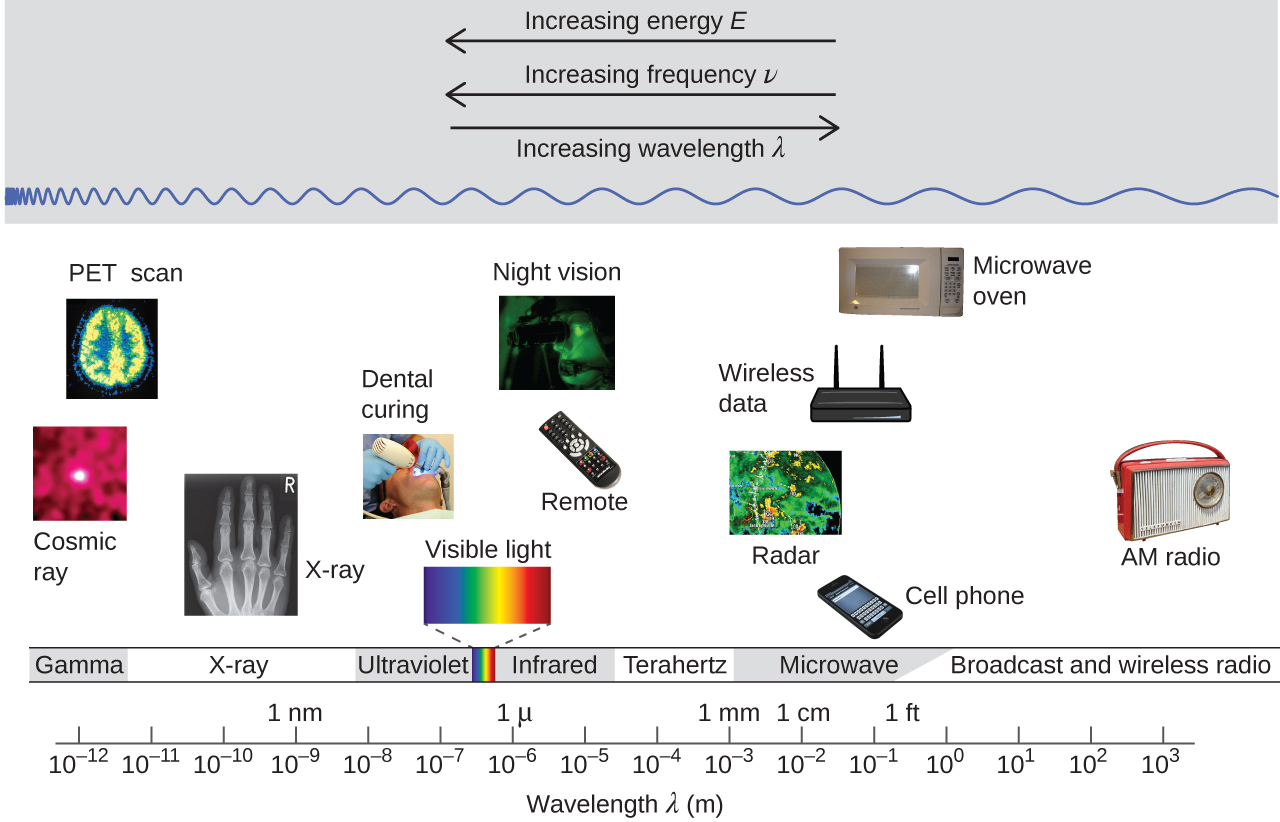Figure 2. Portions of the electromagnetic spectrum are shown in order of decreasing frequency and increasing wavelength. Examples of some applications for various wavelengths include positron emission tomography (PET) scans, X-ray imaging, remote controls, wireless Internet, cellular telephones, and radios. (credit “Cosmic ray”: modification of work by NASA; credit “PET scan”: modification of work by the National Institute of Health; credit “X-ray”: modification of work by Dr. Jochen Lengerke; credit “Dental curing”: modification of work by the Department of the Navy; credit “Night vision”: modification of work by the Department of the Army; credit “Remote”: modification of work by Emilian Robert Vicol; credit “Cell phone”: modification of work by Brett Jordan; credit “Microwave oven”: modification of work by Billy Mabray; credit “Ultrasound”: modification of work by Jane Whitney; credit “AM radio”: modification of work by Dave Clausen)

### Example 1

Determining the Frequency and Wavelength of Radiation
A sodium streetlight gives off yellow light that has a wavelength of 589 nm (1 nm = 1 × 10−9 m). What is the frequency of this light?

Solution
We can rearrange the equation c = λν to solve for the frequency:

$\nu = \frac{c}{\lambda}$

Since c is expressed in meters per second, we must also convert 589 nm to meters.

$\nu = (\frac{2.998 \times 10^8 \;\rule[0.25ex]{0.5em}{0.1ex}\hspace{-0.5em}\text{ms}^{-1}}{589 \;\rule[0.25ex]{1em}{0.1ex}\hspace{-1em}\text{nm}})(\frac{1 \times 10^9 \;\rule[0.25ex]{1em}{0.1ex}\hspace{-1em}\text{nm}}{1\;\rule[0.25ex]{0.5em}{0.1ex}\hspace{-0.5em}\text{m}}) = 5.09 \times 10^{14}\text{s}^{-1}$

One of the frequencies used to transmit and receive cellular telephone signals in the United States is 850 MHz. What is the wavelength in meters of these radio waves?

0.353 m = 35.3 cm

### Wireless CommunicationFigure 3. Radio and cell towers are typically used to transmit long-wavelength electromagnetic radiation. Increasingly, cell towers are designed to blend in with the landscape, as with the Tucson, Arizona, cell tower (right) disguised as a palm tree. (credit left: modification of work by Sir Mildred Pierce; credit middle: modification of work by M.O. Stevens)

Many valuable technologies operate in the radio (3 kHz-300 GHz) frequency region of the electromagnetic spectrum. At the low frequency (low energy, long wavelength) end of this region are AM (amplitude modulation) radio signals (540-2830 kHz) that can travel long distances. FM (frequency modulation) radio signals are used at higher frequencies (87.5-108.0 MHz). In AM radio, the information is transmitted by varying the amplitude of the wave (Figure 4). In FM radio, by contrast, the amplitude is constant and the instantaneous frequency varies.

Other technologies also operate in the radio-wave portion of the electromagnetic spectrum. For example, 4G cellular telephone signals are approximately 880 MHz, while Global Positioning System (GPS) signals operate at 1.228 and 1.575 GHz, local area wireless technology (Wi-Fi) networks operate at 2.4 to 5 GHz, and highway toll sensors operate at 5.8 GHz. The frequencies associated with these applications are convenient because such waves tend not to be absorbed much by common building materials.

One particularly characteristic phenomenon of waves results when two or more waves come into contact: They interfere with each other. Figure 5 shows the interference patterns that arise when light passes through narrow slits closely spaced about a wavelength apart. The fringe patterns produced depend on the wavelength, with the fringes being more closely spaced for shorter wavelength light passing through a given set of slits. When the light passes through the two slits, each slit effectively acts as a new source, resulting in two closely spaced waves coming into contact at the detector (the camera in this case). The dark regions in Figure 5 correspond to regions where the peaks for the wave from one slit happen to coincide with the troughs for the wave from the other slit (destructive interference), while the brightest regions correspond to the regions where the peaks for the two waves (or their two troughs) happen to coincide (constructive interference). Likewise, when two stones are tossed close together into a pond, interference patterns are visible in the interactions between the waves produced by the stones. Such interference patterns cannot be explained by particles moving according to the laws of classical mechanics.Figure 5. Interference fringe patterns are shown for light passing through two closely spaced, narrow slits. The spacing of the fringes depends on the wavelength, with the fringes being more closely spaced for the shorter-wavelength blue light. (credit: PASCO)

### Dorothy Hodgkin

Because the wavelengths of X-rays (10-10,000 picometers [pm]) are comparable to the size of atoms, X-rays can be used to determine the structure of molecules. When a beam of X-rays is passed through molecules packed together in a crystal, the X-rays collide with the electrons and scatter. Constructive and destructive interference of these scattered X-rays creates a specific diffraction pattern. Calculating backward from this pattern, the positions of each of the atoms in the molecule can be determined very precisely. One of the pioneers who helped create this technology was Dorothy Crowfoot Hodgkin.

She was born in Cairo, Egypt, in 1910, where her British parents were studying archeology. Even as a young girl, she was fascinated with minerals and crystals. When she was a student at Oxford University, she began researching how X-ray crystallography could be used to determine the structure of biomolecules. She invented new techniques that allowed her and her students to determine the structures of vitamin B12, penicillin, and many other important molecules. Diabetes, a disease that affects 382 million people worldwide, involves the hormone insulin. Hodgkin began studying the structure of insulin in 1934, but it required several decades of advances in the field before she finally reported the structure in 1969. Understanding the structure has led to better understanding of the disease and treatment options.

Not all waves are travelling waves. Standing waves (also known as stationary waves) remain constrained within some region of space. As we shall see, standing waves play an important role in our understanding of the electronic structure of atoms and molecules. The simplest example of a standing wave is a one-dimensional wave associated with a vibrating string that is held fixed at its two end points. Figure 6 shows the four lowest-energy standing waves (the fundamental wave and the lowest three harmonics) for a vibrating string at a particular amplitude. Although the string’s motion lies mostly within a plane, the wave itself is considered to be one dimensional, since it lies along the length of the string. The motion of string segments in a direction perpendicular to the string length generates the waves and so the amplitude of the waves is visible as the maximum displacement of the curves seen in Figure 6. The key observation from the figure is that only those waves having an integer number, n, of half-wavelengths between the end points can form. A system with fixed end points such as this restricts the number and type of the possible waveforms. This is an example of quantization, in which only discrete values from a more general set of continuous values of some property are observed. Another important observation is that the harmonic waves (those waves displaying more than one-half wavelength) all have one or more points between the two end points that are not in motion. These special points are nodes. The energies of the standing waves with a given amplitude in a vibrating string increase with the number of half-wavelengths n. Since the number of nodes is n – 1, the energy can also be said to depend on the number of nodes, generally increasing as the number of nodes increases.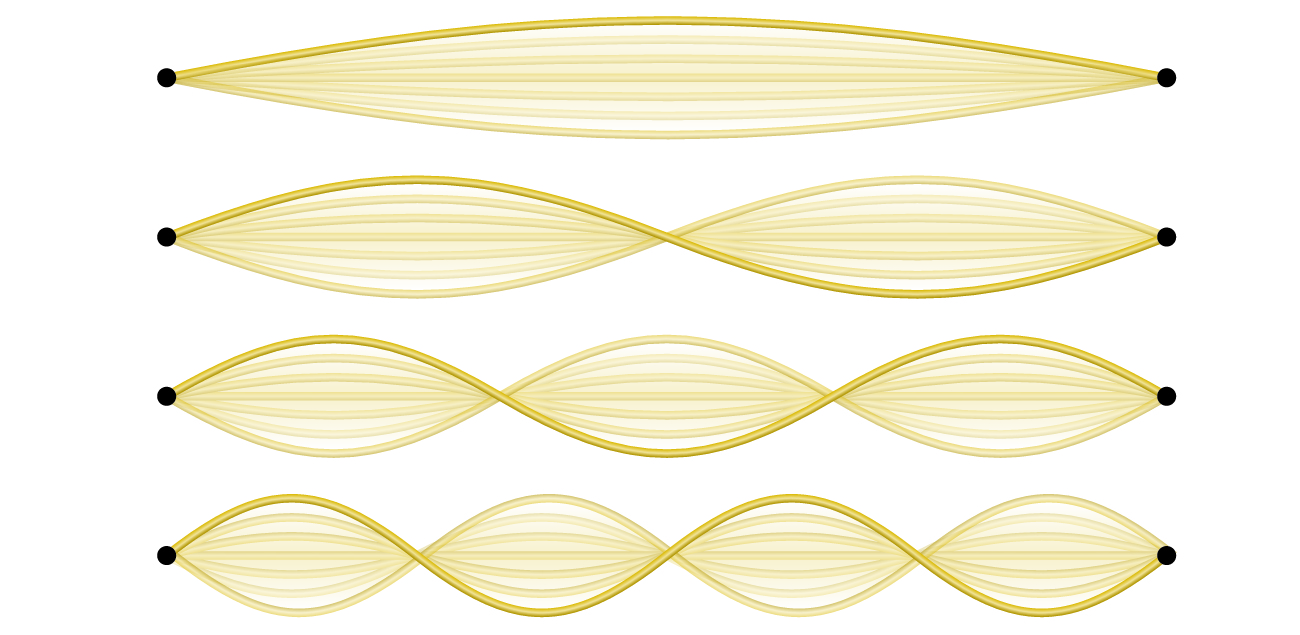Figure 6. A vibrating string shows some one-dimensional standing waves. Since the two end points of the string are held fixed, only waves having an integer number of half-wavelengths can form. The points on the string between the end points that are not moving are called the nodes.

An example of two-dimensional standing waves is shown in Figure 7, which shows the vibrational patterns on a flat surface. Although the vibrational amplitudes cannot be seen like they could in the vibrating string, the nodes have been made visible by sprinkling the drum surface with a powder that collects on the areas of the surface that have minimal displacement. For one-dimensional standing waves, the nodes were points on the line, but for two-dimensional standing waves, the nodes are lines on the surface (for three-dimensional standing waves, the nodes are two-dimensional surfaces within the three-dimensional volume). Because of the circular symmetry of the drum surface, its boundary conditions (the drum surface being tightly constrained to the circumference of the drum) result in two types of nodes: radial nodes that sweep out all angles at constant radii and, thus, are seen as circles about the center, and angular nodes that sweep out all radii at constant angles and, thus, are seen as lines passing through the center. The upper left image in Figure 7 shows two radial nodes, while the image in the lower right shows the vibrational pattern associated with three radial nodes and two angular nodes.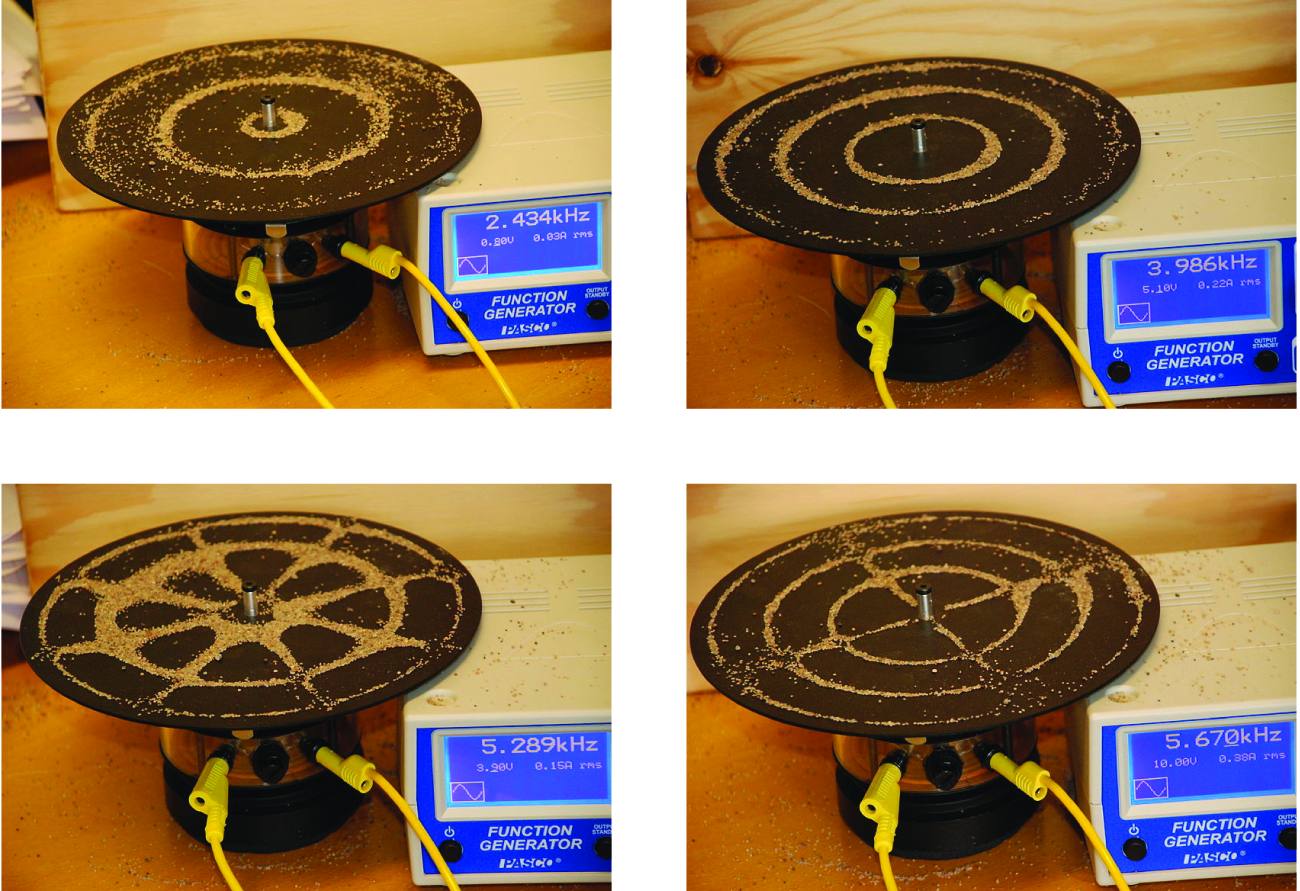Figure 7. Two-dimensional standing waves can be visualized on a vibrating surface. The surface has been sprinkled with a powder that collects near the nodal lines. There are two types of nodes visible: radial nodes (circles) and angular nodes (radii).You can watch the formation of various radial nodes here as singer Imogen Heap projects her voice across a kettle drum.

# Blackbody Radiation and the Ultraviolet Catastrophe

The last few decades of the nineteenth century witnessed intense research activity in commercializing newly discovered electric lighting. This required obtaining a better understanding of the distributions of light emitted from various sources being considered. Artificial lighting is usually designed to mimic natural sunlight within the limitations of the underlying technology. Such lighting consists of a range of broadly distributed frequencies that form a continuous spectrum. Figure 8 shows the wavelength distribution for sunlight. The most intense radiation is in the visible region, with the intensity dropping off rapidly for shorter wavelength ultraviolet (UV) light, and more slowly for longer wavelength infrared (IR) light.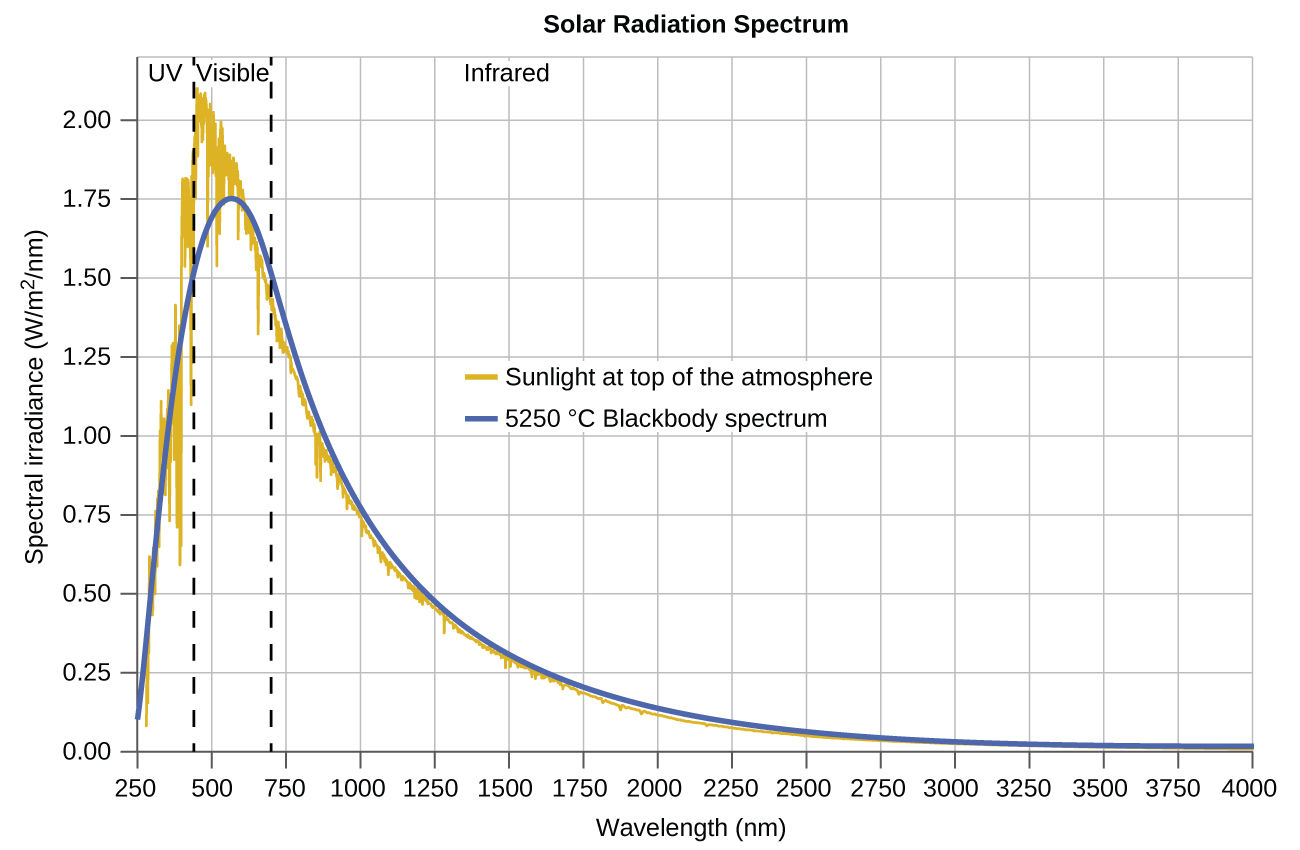Figure 8. The spectral distribution (light intensity vs. wavelength) of sunlight reaches the Earth’s atmosphere as UV light, visible light, and IR light. The unabsorbed sunlight at the top of the atmosphere has a distribution that approximately matches the theoretical distribution of a blackbody at 5250 °C, represented by the blue curve. (credit: modification of work by American Society for Testing and Materials (ASTM) Terrestrial Reference Spectra for Photovoltaic Performance Evaluation)

In Figure 8, the solar distribution is compared to a representative distribution, called a blackbody spectrum, that corresponds to a temperature of 5250 °C. The blackbody spectrum matches the solar spectrum quite well. A blackbody is a convenient, ideal emitter that approximates the behavior of many materials when heated. It is “ideal” in the same sense that an ideal gas is a convenient, simple representation of real gases that works well, provided that the pressure is not too high nor the temperature too low. A good approximation of a blackbody that can be used to observe blackbody radiation is a metal oven that can be heated to very high temperatures. The oven has a small hole allowing for the light being emitted within the oven to be observed with a spectrometer so that the wavelengths and their intensities can be measured. Figure 9 shows the resulting curves for some representative temperatures. Each distribution depends only on a single parameter: the temperature. The maxima in the blackbody curves, λmax, shift to shorter wavelengths as the temperature increases, reflecting the observation that metals being heated to high temperatures begin to glow a darker red that becomes brighter as the temperature increases, eventually becoming white hot at very high temperatures as the intensities of all of the visible wavelengths become appreciable. This common observation was at the heart of the first paradox that showed the fundamental limitations of classical physics that we will examine.

Physicists derived mathematical expressions for the blackbody curves using well-accepted concepts from the theories of classical mechanics and classical electromagnetism. The theoretical expressions as functions of temperature fit the observed experimental blackbody curves well at longer wavelengths, but showed significant discrepancies at shorter wavelengths. Not only did the theoretical curves not show a peak, they absurdly showed the intensity becoming infinitely large as the wavelength became smaller, which would imply that everyday objects at room temperature should be emitting large amounts of UV light. This became known as the “ultraviolet catastrophe” because no one could find any problems with the theoretical treatment that could lead to such unrealistic short-wavelength behavior. Finally, around 1900, Max Planck derived a theoretical expression for blackbody radiation that fit the experimental observations exactly (within experimental error). Planck developed his theoretical treatment by extending the earlier work that had been based on the premise that the atoms composing the oven vibrated at increasing frequencies (or decreasing wavelengths) as the temperature increased, with these vibrations being the source of the emitted electromagnetic radiation. But where the earlier treatments had allowed the vibrating atoms to have any energy values obtained from a continuous set of energies (perfectly reasonable, according to classical physics), Planck found that by restricting the vibrational energies to discrete values for each frequency, he could derive an expression for blackbody radiation that correctly had the intensity dropping rapidly for the short wavelengths in the UV region.

$E = nh\nu$, n = 1, 2, 3, …

The quantity h is a constant now known as Planck’s constant, in his honor. Although Planck was pleased he had resolved the blackbody radiation paradox, he was disturbed that to do so, he needed to assume the vibrating atoms required quantized energies, which he was unable to explain. The value of Planck’s constant is very small, 6.626 × 10−34 joule seconds (J s), which helps explain why energy quantization had not been observed previously in macroscopic phenomena.

# The Photoelectric Effect

The next paradox in the classical theory to be resolved concerned the photoelectric effect (Figure 10). It had been observed that electrons could be ejected from the clean surface of a metal when light having a frequency greater than some threshold frequency was shone on it. Surprisingly, the kinetic energy of the ejected electrons did not depend on the brightness of the light, but increased with increasing frequency of the light. Since the electrons in the metal had a certain amount of binding energy keeping them there, the incident light needed to have more energy to free the electrons. According to classical wave theory, a wave’s energy depends on its intensity (which depends on its amplitude), not its frequency. One part of these observations was that the number of electrons ejected within in a given time period was seen to increase as the brightness increased. In 1905, Albert Einstein was able to resolve the paradox by incorporating Planck’s quantization findings into the discredited particle view of light (Einstein actually won his Nobel prize for this work, and not for his theories of relativity for which he is most famous).

Einstein argued that the quantized energies that Planck had postulated in his treatment of blackbody radiation could be applied to the light in the photoelectric effect so that the light striking the metal surface should not be viewed as a wave, but instead as a stream of particles (later called photons) whose energy depended on their frequency, according to Planck’s formula, E = (or, in terms of wavelength using c = νλ, $E = \frac{hc}{\lambda}$). Electrons were ejected when hit by photons having sufficient energy (a frequency greater than the threshold). The greater the frequency, the greater the kinetic energy imparted to the escaping electrons by the collisions. Einstein also argued that the light intensity did not depend on the amplitude of the incoming wave, but instead corresponded to the number of photons striking the surface within a given time period. This explains why the number of ejected electrons increased with increasing brightness, since the greater the number of incoming photons, the greater the likelihood that they would collide with some of the electrons.

With Einstein’s findings, the nature of light took on a new air of mystery. Although many light phenomena could be explained either in terms of waves or particles, certain phenomena, such as the interference patterns obtained when light passed through a double slit, were completely contrary to a particle view of light, while other phenomena, such as the photoelectric effect, were completely contrary to a wave view of light. Somehow, at a deep fundamental level still not fully understood, light is both wavelike and particle-like. This is known as wave-particle duality.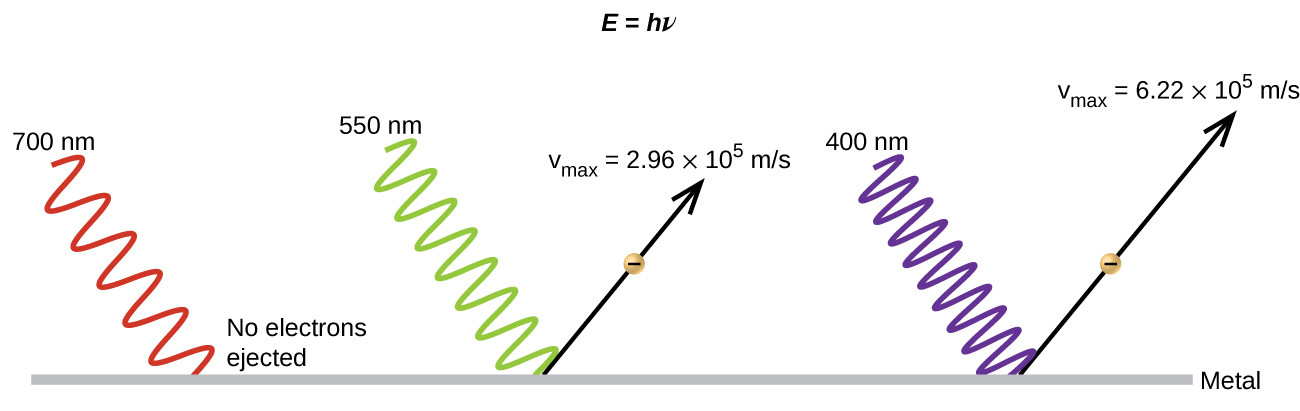Figure 10. Photons with low frequencies do not have enough energy to cause electrons to be ejected via the photoelectric effect. For any frequency of light above the threshold frequency, the kinetic energy of ejected electron will be proportional to the energy of the incoming photon.

### Example 2

When we see light from a neon sign, we are observing radiation from excited neon atoms. If this radiation has a wavelength of 640 nm, what is the energy of the photon being emitted?

Solution
We use the part of Planck’s equation that includes the wavelength, λ, and convert units of nanometers to meters so that the units of λ and c are the same.

$\begin{array}{l} E = \frac{hc}{\lambda} \\[1em] E = \frac{(6.626 \times 10^{-34}\text{J}\rule[0.4ex]{0.35em}{0.1ex}\hspace{-0.35em}\text{s})(2.998 \times 10^8 \text{m}\rule[0.4ex]{0.35em}{0.1ex}\hspace{-0.35em}\text{s}^{-1})}{(640 \;\rule[0.5ex]{1em}{0.1ex}\hspace{-1em}\text{nm})(\frac{1 \;\text{m}}{10^9 \;\rule[0.25ex]{0.8em}{0.1ex}\hspace{-0.8em}\text{nm}})} \\[1em] E = 3.10 \times 10^{-19} \text{J} \end{array}$

The microwaves in an oven are of a specific frequency that will heat the water molecules contained in food. (This is why most plastics and glass do not become hot in a microwave oven-they do not contain water molecules.) This frequency is about 3 × 109 Hz. What is the energy of one photon in these microwaves?

2 × 10−24 JUse this simulation program to experiment with the photoelectric effect to see how intensity, frequency, type of metal, and other factors influence the ejected photons.

### Example 3

Photoelectric Effect
Identify which of the following statements are false and, where necessary, change the italicized word or phrase to make them true, consistent with Einstein’s explanation of the photoelectric effect.

(a) Increasing the brightness of incoming light increases the kinetic energy of the ejected electrons.

(b) Increasing the wavelength of incoming light increases the kinetic energy of the ejected electrons.

(c) Increasing the brightness of incoming light increases the number of ejected electrons.

(d) Increasing the frequency of incoming light can increase the number of ejected electrons.

Solution
(a) False. Increasing the brightness of incoming light has no effect on the kinetic energy of the ejected electrons. Only energy, not the number or amplitude, of the photons influences the kinetic energy of the electrons.

(b) False. Increasing the frequency of incoming light increases the kinetic energy of the ejected electrons. Frequency is proportional to energy and inversely proportional to wavelength. Frequencies above the threshold value transfer the excess energy into the kinetic energy of the electrons.

(c) True. Because the number of collisions with photons increases with brighter light, the number of ejected electrons increases.

(d) True with regard to the threshold energy binding the electrons to the metal. Below this threshold, electrons are not emitted and above it they are. Once over the threshold value, further increasing the frequency does not increase the number of ejected electrons

Calculate the threshold energy in kJ/mol of electrons in aluminum, given that the lowest frequency photon for which the photoelectric effect is observed is 9.87 × 1014 Hz.

3.94 × 105 kJ/mol

# Line Spectra

Another paradox within the classical electromagnetic theory that scientists in the late nineteenth century struggled with concerned the light emitted from atoms and molecules. When solids, liquids, or condensed gases are heated sufficiently, they radiate some of the excess energy as light. Photons produced in this manner have a range of energies, and thereby produce a continuous spectrum in which an unbroken series of wavelengths is present. Most of the light generated from stars (including our sun) is produced in this fashion. You can see all the visible wavelengths of light present in sunlight by using a prism to separate them. As can be seen in Figure 8, sunlight also contains UV light (shorter wavelengths) and IR light (longer wavelengths) that can be detected using instruments but that are invisible to the human eye. Incandescent (glowing) solids such as tungsten filaments in incandescent lights also give off light that contains all wavelengths of visible light. These continuous spectra can often be approximated by blackbody radiation curves at some appropriate temperature, such as those shown in Figure 9.

In contrast to continuous spectra, light can also occur as discrete or line spectra having very narrow line widths interspersed throughout the spectral regions such as those shown in Figure 12. Exciting a gas at low partial pressure using an electrical current, or heating it, will produce line spectra. Fluorescent light bulbs and neon signs operate in this way (Figure 11). Each element displays its own characteristic set of lines, as do molecules, although their spectra are generally much more complicated.Figure 11. Neon signs operate by exciting a gas at low partial pressure using an electrical current. This sign shows the elaborate artistic effects that can be achieved. (credit: Dave Shaver)

Each emission line consists of a single wavelength of light, which implies that the light emitted by a gas consists of a set of discrete energies. For example, when an electric discharge passes through a tube containing hydrogen gas at low pressure, the H2 molecules are broken apart into separate H atoms and we see a blue-pink color. Passing the light through a prism produces a line spectrum, indicating that this light is composed of photons of four visible wavelengths, as shown in Figure 12.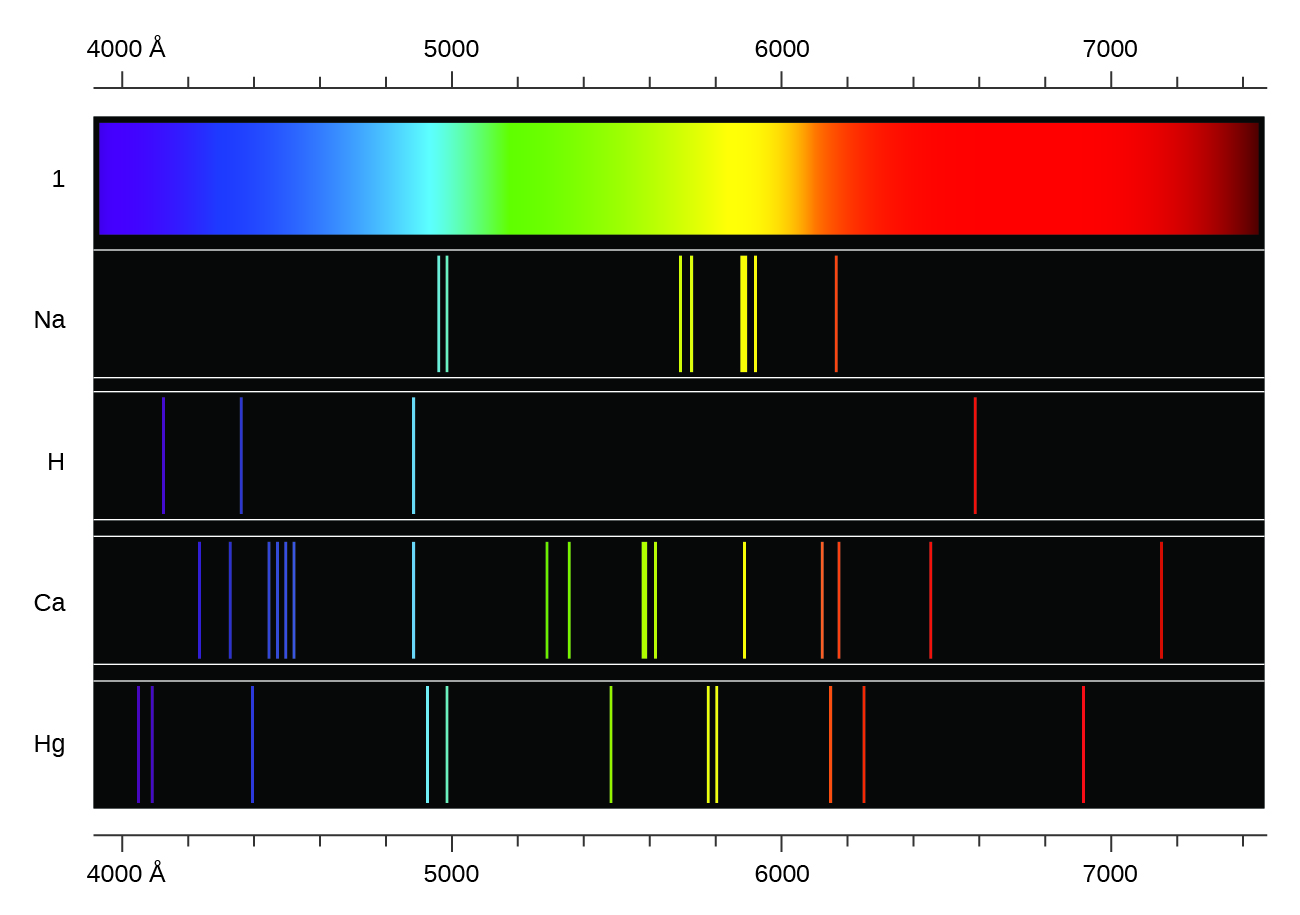Figure 12. Compare the two types of emission spectra: continuous spectrum of white light (top) and the line spectra of the light from excited sodium, hydrogen, calcium, and mercury atoms.

The origin of discrete spectra in atoms and molecules was extremely puzzling to scientists in the late nineteenth century, since according to classical electromagnetic theory, only continuous spectra should be observed. Even more puzzling, in 1885, Johann Balmer was able to derive an empirical equation that related the four visible wavelengths of light emitted by hydrogen atoms to whole integers. That equation is the following one, in which k is a constant:

$\frac{1}{\lambda} = k(\frac{1}{4} - \frac{1}{n^2}), n = 3, 4, 5, 6$

Other discrete lines for the hydrogen atom were found in the UV and IR regions. Johannes Rydberg generalized Balmer’s work and developed an empirical formula that predicted all of hydrogen’s emission lines, not just those restricted to the visible range, where, n1 and n2 are integers, n1 < n2, and R is the Rydberg constant (1.097 × 107 m−1).

$\frac{1}{\lambda} = R_{\infty} (\frac{1}{n^2_1} - \frac{1}{n^2_2})$

Even in the late nineteenth century, spectroscopy was a very precise science, and so the wavelengths of hydrogen were measured to very high accuracy, which implied that the Rydberg constant could be determined very precisely as well. That such a simple formula as the Rydberg formula could account for such precise measurements seemed astounding at the time, but it was the eventual explanation for emission spectra by Neils Bohr in 1913 that ultimately convinced scientists to abandon classical physics and spurred the development of modern quantum mechanics.

# Key Concepts and Summary

Light and other forms of electromagnetic radiation move through a vacuum with a constant speed, c, of 2.998 × 108 m s−1. This radiation shows wavelike behavior, which can be characterized by a frequency, ν, and a wavelength, λ, such that c = λν. Light is an example of a travelling wave. Other important wave phenomena include standing waves, periodic oscillations, and vibrations. Standing waves exhibit quantization, since their wavelengths are limited to discrete integer multiples of some characteristic lengths. Electromagnetic radiation that passes through two closely spaced narrow slits having dimensions roughly similar to the wavelength will show an interference pattern that is a result of constructive and destructive interference of the waves. Electromagnetic radiation also demonstrates properties of particles called photons. The energy of a photon is related to the frequency (or alternatively, the wavelength) of the radiation as E = (or $E = \frac{hc}{\lambda}$), where h is Planck’s constant. That light demonstrates both wavelike and particle-like behavior is known as wave-particle duality. All forms of electromagnetic radiation share these properties, although various forms including X-rays, visible light, microwaves, and radio waves interact differently with matter and have very different practical applications. Electromagnetic radiation can be generated by exciting matter to higher energies, such as by heating it. The emitted light can be either continuous (incandescent sources like the sun) or discrete (from specific types of excited atoms). Continuous spectra often have distributions that can be approximated as blackbody radiation at some appropriate temperature. The line spectrum of hydrogen can be obtained by passing the light from an electrified tube of hydrogen gas through a prism. This line spectrum was simple enough that an empirical formula called the Rydberg formula could be derived from the spectrum. Three historically important paradoxes from the late 19th and early 20th centuries that could not be explained within the existing framework of classical mechanics and classical electromagnetism were the blackbody problem, the photoelectric effect, and the discrete spectra of atoms. The resolution of these paradoxes ultimately led to quantum theories that superseded the classical theories.

# Key Equations

• c = λν
• $E = h\nu = \frac{hc}{\lambda}$, where h = 6.626 × 10−34 J s
• $\frac{1}{\lambda} = R_{\infty} (\frac{1}{n^2_1} - \frac{1}{n^2_2})$

### Chemistry End of Chapter Exercises

1. The light produced by a red neon sign is due to the emission of light by excited neon atoms. Qualitatively describe the spectrum produced by passing light from a neon lamp through a prism.
2. An FM radio station found at 103.1 on the FM dial broadcasts at a frequency of 1.031 × 108 s−1 (103.1 MHz). What is the wavelength of these radio waves in meters?
3. FM-95, an FM radio station, broadcasts at a frequency of 9.51 × 107 s−1 (95.1 MHz). What is the wavelength of these radio waves in meters?
4. A bright violet line occurs at 435.8 nm in the emission spectrum of mercury vapor. What amount of energy, in joules, must be released by an electron in a mercury atom to produce a photon of this light?
5. Light with a wavelength of 614.5 nm looks orange. What is the energy, in joules, per photon of this orange light? What is the energy in eV (1 eV = 1.602 × 10−19 J)?
6. Heated lithium atoms emit photons of light with an energy of 2.961 × 10−19 J. Calculate the frequency and wavelength of one of these photons. What is the total energy in 1 mole of these photons? What is the color of the emitted light?
7. A photon of light produced by a surgical laser has an energy of 3.027 × 10−19 J. Calculate the frequency and wavelength of the photon. What is the total energy in 1 mole of photons? What is the color of the emitted light?
8. When rubidium ions are heated to a high temperature, two lines are observed in its line spectrum at wavelengths (a) 7.9 × 10−7 m and (b) 4.2 × 10−7 m. What are the frequencies of the two lines? What color do we see when we heat a rubidium compound?
9. The emission spectrum of cesium contains two lines whose frequencies are (a) 3.45 × 1014 Hz and (b) 6.53 × 1014 Hz. What are the wavelengths and energies per photon of the two lines? What color are the lines?
10. Photons of infrared radiation are responsible for much of the warmth we feel when holding our hands before a fire. These photons will also warm other objects. How many infrared photons with a wavelength of 1.5 × 10−6 m must be absorbed by the water to warm a cup of water (175 g) from 25.0 °C to 40 °C?
11. One of the radiographic devices used in a dentist’s office emits an X-ray of wavelength 2.090 × 10−11 m. What is the energy, in joules, and frequency of this X-ray?
12. The eyes of certain reptiles pass a single visual signal to the brain when the visual receptors are struck by photons of a wavelength of 850 nm. If a total energy of 3.15 × 10−14 J is required to trip the signal, what is the minimum number of photons that must strike the receptor?
13. RGB color television and computer displays use cathode ray tubes that produce colors by mixing red, green, and blue light. If we look at the screen with a magnifying glass, we can see individual dots turn on and off as the colors change. Using a spectrum of visible light, determine the approximate wavelength of each of these colors. What is the frequency and energy of a photon of each of these colors?

(a) The laser on a Blu-ray player has a wavelength of 405 nm. In what region of the electromagnetic spectrum is this radiation? What is its frequency?

(b) A Blu-ray laser has a power of 5 milliwatts (1 watt = 1 J s−1). How many photons of light are produced by the laser in 1 hour?

(c) The ideal resolution of a player using a laser (such as a Blu-ray player), which determines how close together data can be stored on a compact disk, is determined using the following formula: Resolution = 0.60(λ/NA), where λ is the wavelength of the laser and NA is the numerical aperture. Numerical aperture is a measure of the size of the spot of light on the disk; the larger the NA, the smaller the spot. In a typical Blu-ray system, NA = 0.95. If the 405-nm laser is used in a Blu-ray player, what is the closest that information can be stored on a Blu-ray disk?

(d) The data density of a Blu-ray disk using a 405-nm laser is 1.5 × 107 bits mm−2. Disks have an outside diameter of 120 mm and a hole of 15-mm diameter. How many data bits can be contained on the disk? If a Blu-ray disk can hold 9,400,000 pages of text, how many data bits are needed for a typed page? (Hint: Determine the area of the disk that is available to hold data. The area inside a circle is given by A = πr2, where the radius r is one-half of the diameter.)

15. What is the threshold frequency for sodium metal if a photon with frequency 6.66 × 1014 s−1 ejects an electron with 7.74 × 10−20 J kinetic energy? Will the photoelectric effect be observed if sodium is exposed to orange light?

## Glossary

amplitude
extent of the displacement caused by a wave (for sinusoidal waves, it is one-half the difference from the peak height to the trough depth, and the intensity is proportional to the square of the amplitude)
blackbody
idealized perfect absorber of all incident electromagnetic radiation; such bodies emit electromagnetic radiation in characteristic continuous spectra called blackbody radiation
continuous spectrum
electromagnetic radiation given off in an unbroken series of wavelengths (e.g., white light from the sun)
energy transmitted by waves that have an electric-field component and a magnetic-field component
electromagnetic spectrum
range of energies that electromagnetic radiation can comprise, including radio, microwaves, infrared, visible, ultraviolet, X-rays, and gamma rays; since electromagnetic radiation energy is proportional to the frequency and inversely proportional to the wavelength, the spectrum can also be specified by ranges of frequencies or wavelengths
frequency (ν)
number of wave cycles (peaks or troughs) that pass a specified point in space per unit time
hertz (Hz)
the unit of frequency, which is the number of cycles per second, s−1
intensity
property of wave-propagated energy related to the amplitude of the wave, such as brightness of light or loudness of sound
interference pattern
pattern typically consisting of alternating bright and dark fringes; it results from constructive and destructive interference of waves
line spectrum
electromagnetic radiation emitted at discrete wavelengths by a specific atom (or atoms) in an excited state
node
any point of a standing wave with zero amplitude
photon
smallest possible packet of electromagnetic radiation, a particle of light
quantization
occurring only in specific discrete values, not continuous
standing wave
(also, stationary wave) localized wave phenomenon characterized by discrete wavelengths determined by the boundary conditions used to generate the waves; standing waves are inherently quantized
wave
oscillation that can transport energy from one point to another in space
wavelength (λ)
distance between two consecutive peaks or troughs in a wave
wave-particle duality
term used to describe the fact that elementary particles including matter exhibit properties of both particles (including localized position, momentum) and waves (including nonlocalization, wavelength, frequency)

### Solutions

Answers to Chemistry End of Chapter Exercises

1. The spectrum consists of colored lines, at least one of which (probably the brightest) is red.

3. 3.15 m

5. 3.233 × 10−19 J; 2.018 eV

7. ν = 4.568 × 1014 s; λ = 656.3 nm; Energy mol−1 = 1.823 × 105 J mol−1; red

9. (a) λ = 8.69 × 10−7 m; E = 2.29 × 10−19 J; (b) λ = 4.59 × 10−7 m; E = 4.33 × 10−19 J; The color of (a) is red; (b) is blue.

11. E = 9.502 × 10−15 J; ν = 1.434 × 1019 s−1

13. Red: 660 nm; 4.54 × 1014 Hz; 3.01 × 10−19 J. Green: 520 nm; 5.77 × 1014 Hz; 3.82 × 10−19 J. Blue: 440 nm; 6.81 × 1014 Hz; 4.51 × 10−19 J. Somewhat different numbers are also possible.

15. 5.49 × 1014 s−1; no